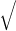# Dimensional Analysis Pendulum Equation

khaledS

## Homework Statement

The period of a simple pendulum, defined as the time necessary for one complete oscillation, is measured in time units and is given by
T = 2πℓ/g
where ℓ is the length of the pendulum and g is the acceleration due to gravity, in units of length divided by time squared. Show that this equation is dimensionally consistent. (You might want to check the formula using your keys at the end of a string and a stopwatch.

## Homework Equations

T=[t] possibly [S] because we are talking about a time period. l=L g=m/s^2 or [L/LT^2]

## The Attempt at a Solution

I am attempting dimensional analysis on this problem but I get lost after a certain point because of the square root and two paths of solving the problem. This is my work thus far and would like insight as to where I am going wrong or what steps I may have missed.
[S]= square root ([m]/[m/s^2] and the m's would cancel and were left with square root of 1/s^2 which becomes [S] so it's dimensionally correct because [S]=[S] and 2 pie is constant so it's irrelevant to the dimensional analysis. Or is not giving me the T=[S] in the problem which would then change it because [t]= square root [L/L/T^2] where L's cancel and are left with square root of 1/T^2 which becomes T so [t]=[t]. Am I doing this correctly?

Last edited by a moderator:

Staff EmeritusYou did that part wrong. You are left with sqrt (T^2) = T. But I think you have the right idea. Just don't cheat!